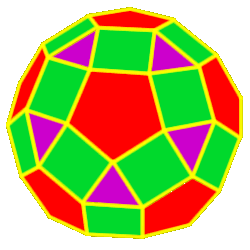# Polyhedra Rhombicosidodecahedron

 The RhombicosidodecahedronThe rhombicosidodecahedron is created by either truncating (cutting off) the dodecahedron one half of the way into each side or by truncating the icosahedron one half of the way into each side 62 total faces: 20 equilateral triangles, 30 squares and 12 regular pentagons 60 vertices: 1 triangle, 2 squares and 1 pentagon 120 edges Dihedral angles:  148 degrees, 17 minutes for the pent-sqr angle and 159 degrees, 6 minutes for the tri-sqr angle